## Force Law for Simple Harmonic Motion

The force acting simple harmonic motion is proportional to the displacement and is always directed towards the centre of motion.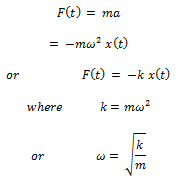Simple harmonic motion is the motion executed by a particle subject to a force, which is proportional to the displacement of the particle and is directed towards the mean position.

Since the force F is proportional to x rather than to some other power of x, such a system is also referred to as a linear harmonic oscillator. Systems in which the restoring force is a nonlinear function of x are termed as non-linear harmonic or an harmonic oscillators.

## Energy in Simple Harmonic Motion

### Total energy in S.H.M:

The energy of a harmonic oscillator is partly kinetic and partly potential. When a body is displaced from its equilibrium position by doing work upon it, it acquires potential energy. When the body is released, it begins to move back with a velocity, thus acquiring kinetic energy.

### Kinetic Energy:

At any instant, the displacement of a particle executing S.H.M. is given by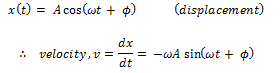Kinetic Energy(K) of such a particle, which is defined as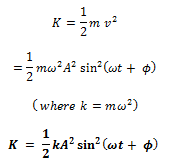### Potential Energy:

When the displacement of a particle from its equilibrium position is x, the restoring force acting on it is,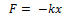If we displace the particle further through a small distance dx, then work done against the restoring force is given by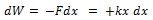Hence the potential energy of a particle at displacement x is given by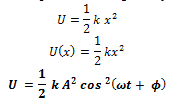### Total Energy:

At any displacement x, the total energy of a harmonic oscillatory is given by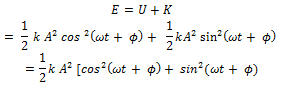The quantity within the square brackets above is unity and we have,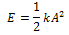The total mechanical energy of a harmonic oscillator is thus independent of time as expected for motion under any conservative force. The time and displacement dependence of the potential and kinetic energies of a linear simple harmonic oscillator are shown in Fig.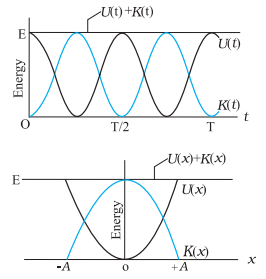Fig. (a) K, U and E as function of time t for a harmonic oscillator. (b) K, U and E as function of displacement x for a harmonic oscillator.

Some systems executing simple Harmonic Motion

In the subsequent part of this section, we discuss the motion executed by some such systems